# Independent Probability

Independent probability – one event DOES NOT affect the outcome of the other.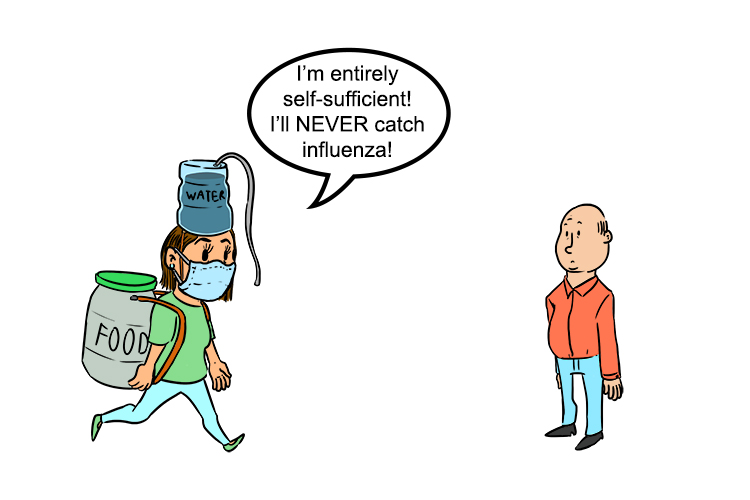This woman is not dependent on anyone else to survive, she will never catch influenza (influence).

Independent probability - NOT Influenced

Example 1

A bag contains 6 marbles, four red and two yellow. A marble is taken from the bag, its colour written down then it is returned to the bag. A marble is again taken from the bag, and its colour written down.

1.  What is the probability of getting two red marbles?

2.  And what is the probability of getting a red and a yellow marble?

Start by drawing a probability tree: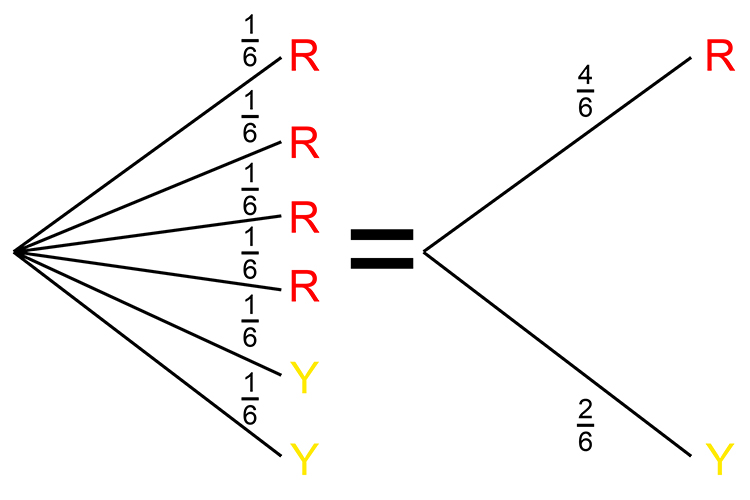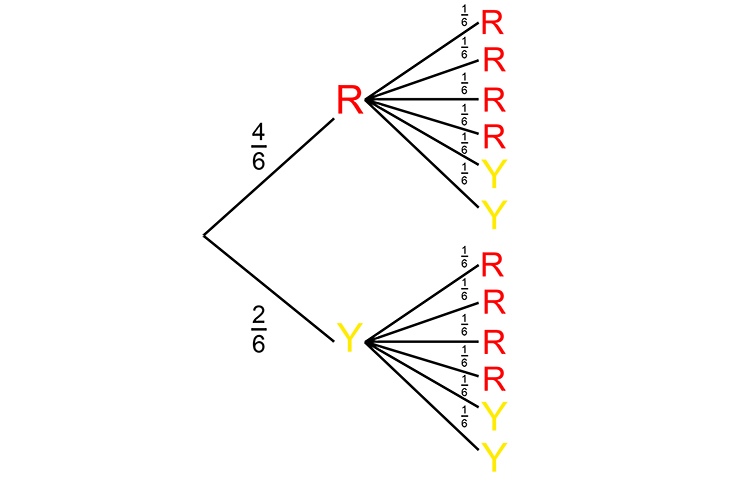This can be redrawn as: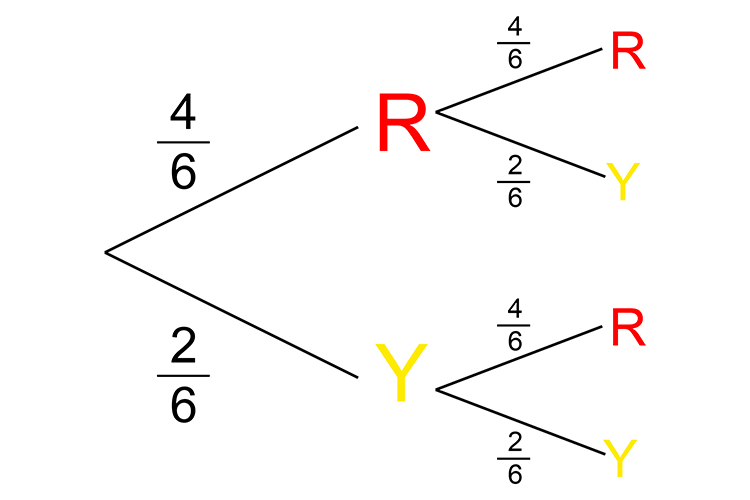This is the probability tree for this example.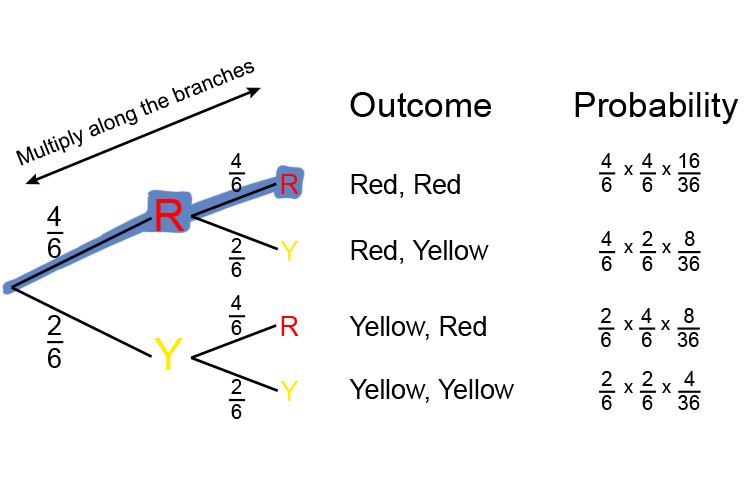Add these up to check they equal 1 16/36+8/36+8/36+4/36=(16+8+8+4)/36=36/36=1

I.  Therefore from the diagram above the probability of getting two red marbles is:

Red, Red =16/36=8/18=4/9

Probability of two reds is 4 in 9 or = 0.44 And if 1 = 100%   then 0.44 = 44% chance.

II. What is the probability of getting a red and a yellow marble?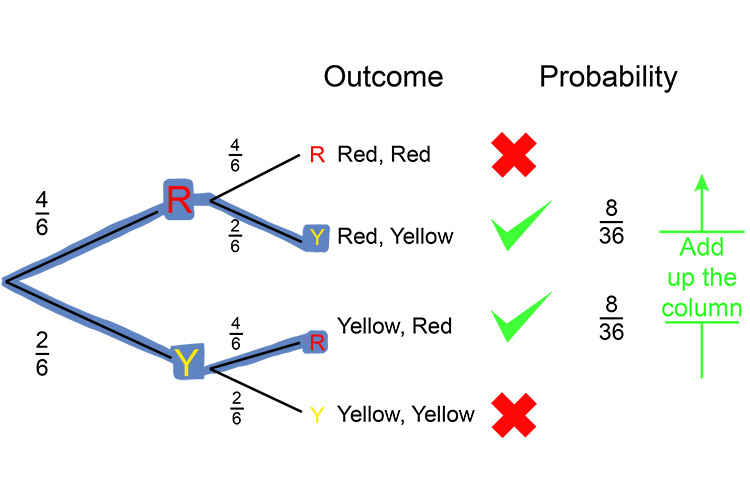8/36+8/36=(8+8)/36=16/36=8/18=4/9